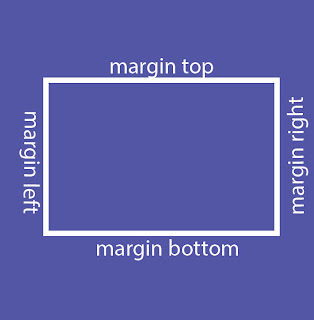## Posts

### How to find the square root in python

Python program to find the square root In this program,you  will about the python program how to find the square root of any number by two method: By using exponent function  By using the cmath module.  For understand the program you have the knowledge: Python input,output. Python data types. Python operators. Example for square root of positive number Code:     # Python Program to calculate the square root for positive number number = 10 number_sqrt = number ** 0.5 print ( 'square root %0.3f is %0.3f' %(number ,number_sqrt)) Output:     square root 10.000 is 3.162 In the above program,value is store in the number variable and then exponent with help ** operator  with power 0.5(½) store in the number_sqrt variable.At the end of the program,display with  help of print function. Example for For real and complex number: Source code: `         import cmath   number = 2 + 2j   number_sqrt = cmath.sqrt(number) print ( 'square root  {0} = {1:0.3f}+{2:0.3f}j'   . format (num

### How to add number in python

Add number in Python To  understand how to add the two number in the python ,first of all you have know how to take input  in python and display output in python . Add the two number Following is the example code for add the two in python: # the following code  adds two numbers by assigning value to variables   number1 = 7 number2 = 4.7   # Add two numbers sum = number1 + number2   # Now Display the sum print ( 'The sum of {0} and {1} is {2}' . format (number1, number2, sum))     Now we will see how add numbers with the help of taking input from the user. Example code: Add the two number by user input    # the following code  taking two number from the user then  add into the sum variable.   number1 = input ( 'Enter first number: ' ) number2 = input ( 'Enter second number: ' )   # Add two numbers sum = float (number1) + float (number2)   # Now Display the sum print ( 'The sum of {0} and {1} is {2}' . format (number1, number2, sum))     In the above exa

### Python program to print Hello wolrd

How to print Hello world! In the python This program is very simple, It is usually used to illustrate the syntax of the specific programming language. To understand this program you have the knowledge of the python basic function of python. Source Code # python program print hello world print('Hello, world!') Output Hello, world! In the above example, we use the built-in print function of python. The string is the sequence of the character, In the python string enclosed in the single ‘’, double” “,  and triple  quotes

### code of Game of Chance

﻿ A Game of Chance One of the most popular games o in casinos and back alleys triangular  games of chance is a dice game known as “craps,”  It is played in hack alleys throughout the world.  Following are rules of the Game: roll two dice. Each die has six faces. These faces contain one, two, three, four, five, and six spots.  the dice  to rest, the sum of the spots on the two upward faces is calculate. If sum is 7 or 11 on the first throw, the player wins.  If the sum is two, three, or twelve on the first throw Called "craps"),  the player loses (mean the "house" wins).  If the sum is four, five, six, eighth, nine, or ten on the first throw, then that sum becomes the player's "point.”  To win, you must continue rolling the dice until you make your points. The player loses by rolling a 7 before making the point.  Code of the game:   #include<stdio.h>  #include<stdlib.h>   #include<time.h>  /*contains prototype for function time */ enum St